# Evaluating piecewise functions

### Evaluating piecewise functions

#### Lessons

Piecewise function:

A function consisted of multiple sub-functions with each applying to a specific interval within the domain of the main function
• 1.
Given the following function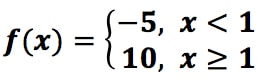a)
evaluate $f$(19)

b)
evaluate $f$(-200)

c)
evaluate $f$(1)

• 2.
Given the following function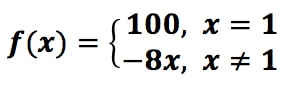a)
evaluate $f$(10)

b)
evaluate $f$(1)

c)
evaluate $f$(-1)

• 3.
For the following function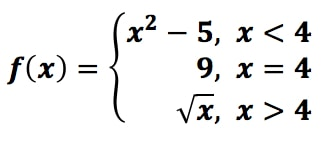a)
evaluate $f$(3)

b)
evaluate $f$(4)

c)
evaluate $f$(16)

• 4.
For the following function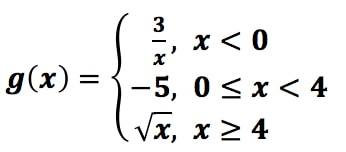a)
evaluate $f$(-9)

b)
evaluate $f$(4)

c)
evaluate $f$(9)

• 5.
For the following function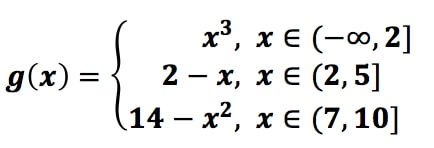a)
evaluate $f$(-100)

b)
evaluate $f$(2)

c)
evaluate $f$(6)

d)
evaluate $f$(10)